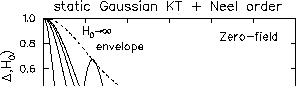Next: References Up: A Appendix Previous: A.2 The 10/3 effect## A.3 Relaxation function in Néel state with randomness

In the true Néel state, a muon spin exhibits a Larmor precession. In a randomly frozen spin system, it exhibits the Gaussian Kubo-Toyabe function. In the following, an intermediate situation between these cases is considered.

If a spin system is frozen almost randomly, but having a non-zero sublattice magnetization (Fig.65), the local field at muon site will be a Gaussian distribution around a static field:

where,is the isotropic Gaussian distribution induced by the randomly frozen spin component andis a well defined static field from the Néel ordered component.Muon spin relaxation in this field distribution is expressed as:

where the integral aboutis taken over the sample geometry. In a polycrystalline sample (, isotropic) , the integral aboutis analytically performed, yielding the muon relaxation as:

Here, the integral overis expressed in polar coordinate. Since the Gaussian distribution is isotropic, the angular part is easily integrated; it gives the 1/3 and 2/3 components. The integral of the radial part is also performed analytically:

In the limit, this function becomes the Gaussian Kubo-Toyabe function (eq.18), and in the large H0 limit, it exhibits a damped oscillation with a frequency associated with H0. In Fig.66, the function obtained is drawn for various H0. A crossover from the Gaussian Kubo-Toyabe behavior to a damped oscillation is shown.Next: References Up: A Appendix Previous: A.2 The 10/3 effect